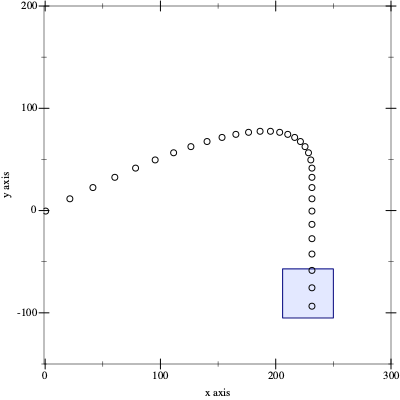# Plotting in a Racket Notebook

::

I recently started experimenting with the Racket notebook package, IRacket. Notebooks are handy for experimentation and visualization, so I wanted to be able to display a plot. I discovered that `(require plot)` did not work, but if I changed that slightly to `(require plot/pict)`, I was able to display a plot in the notebook.

This is an example of plotting in a notebook from Advent of Code 2021 - Day 17:

 ``` 1 2 3 4 5 6 7 8 9 10 11 12 13 14 15 16 17 18 19 20 21 22 23 24 25 26 27``` ```#lang iracket/lang #:require racket (require plot/pict) (define-values (x-max y-min) (values 250 -105)) (define (trajectory dx dy [x 0] [y 0] [ result '() ]) (if (or (> x x-max) (< y y-min)) (reverse result) (let ([ result (cons (list x y) result) ] [ dx (cond [ (> dx 0) (sub1 dx) ] [ else dx ]) ] [ dy (sub1 dy) ] [ x (+ x dx) ] [ y (+ y dy) ]) (trajectory dx dy x y result)))) (define (plot-trajectory dx dy) (let ([ pts (trajectory dx dy) ]) (plot (list (rectangles (list (vector (ivl 206 250) (ivl -57 -105)))) (points pts)) #:x-min -1 #:x-max 300 #:y-min -150 #:y-max 200))) (plot-trajectory 21 12) ```

``````